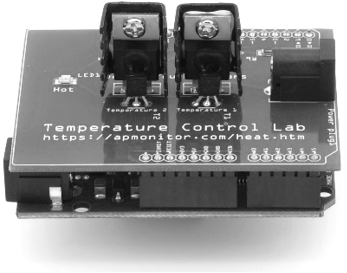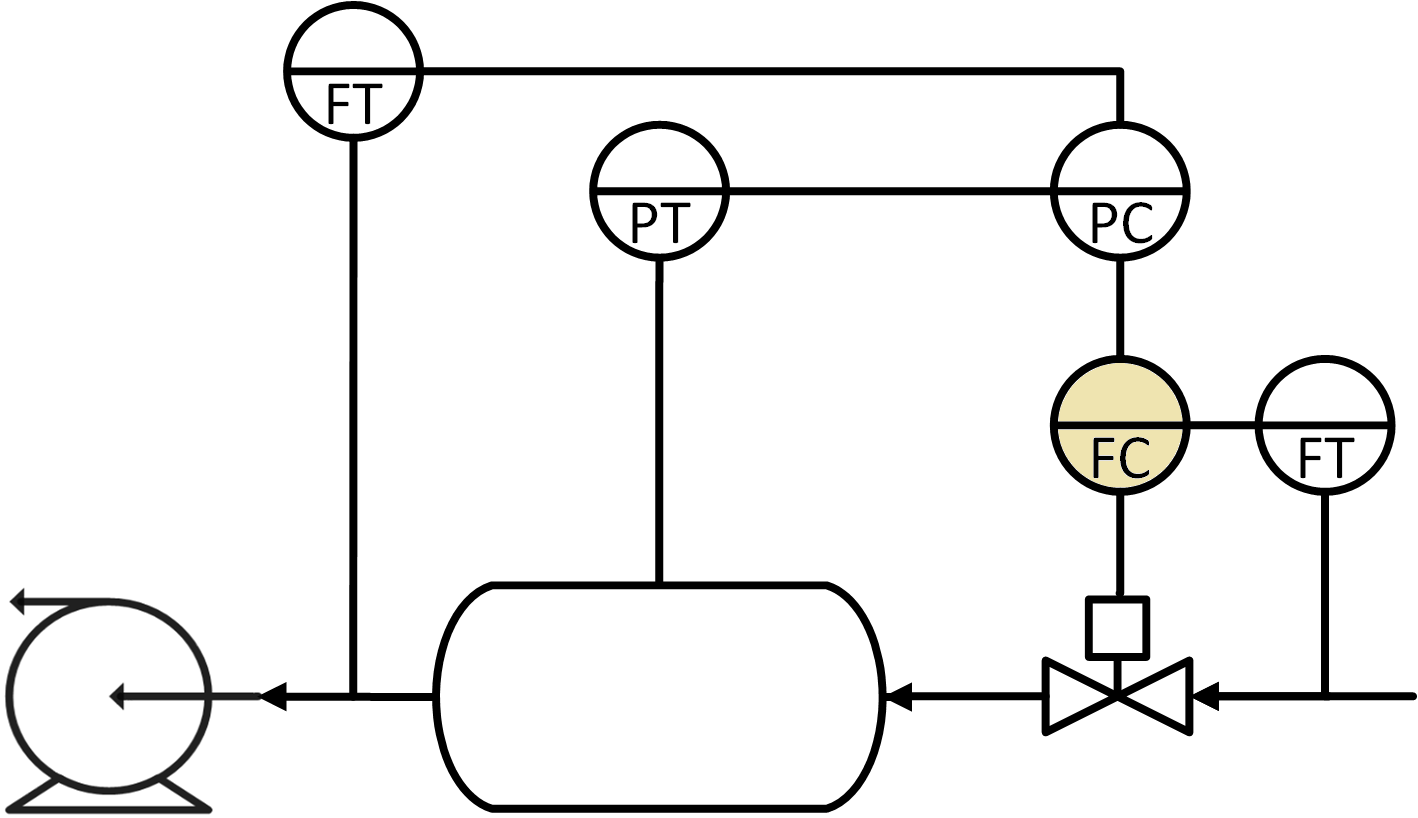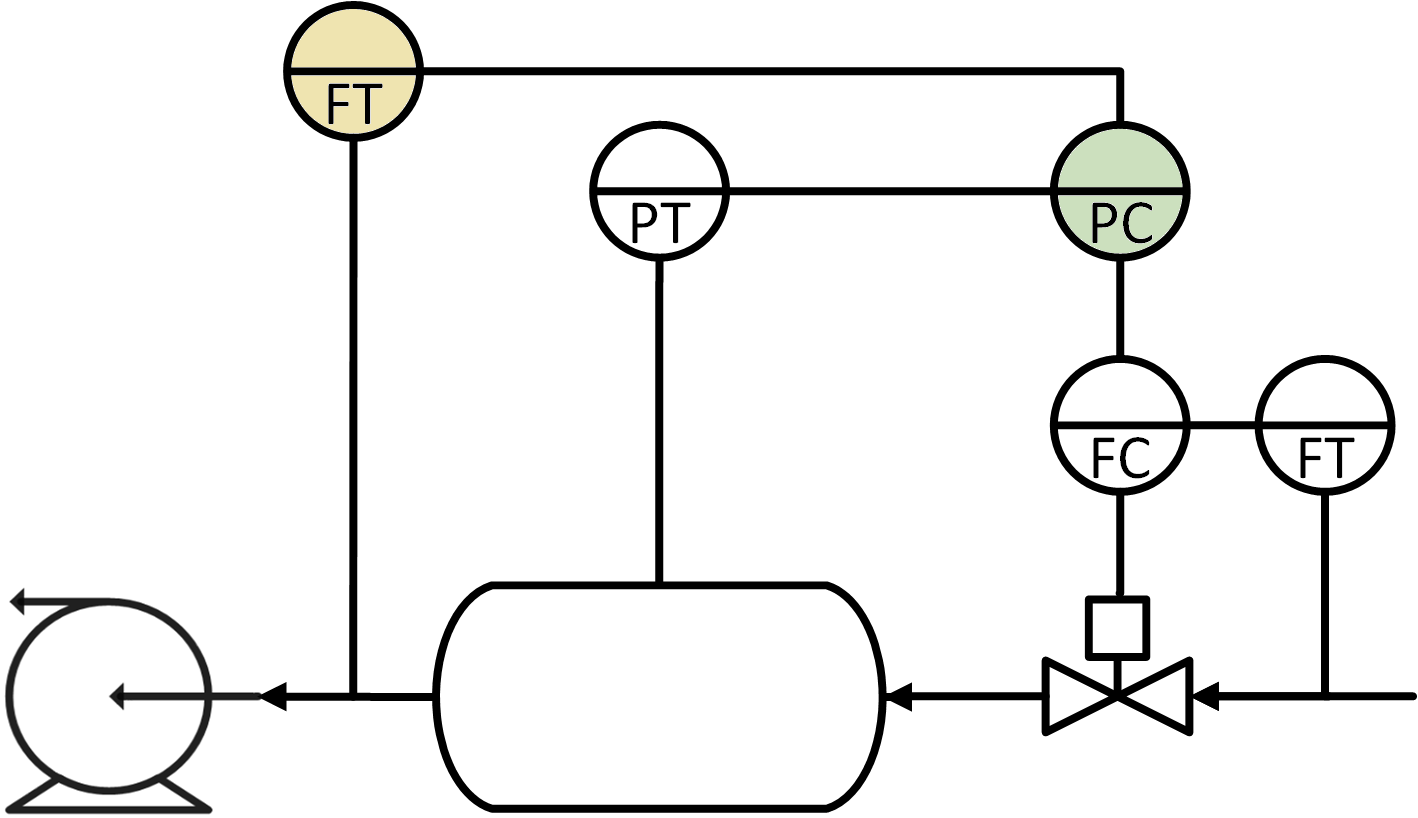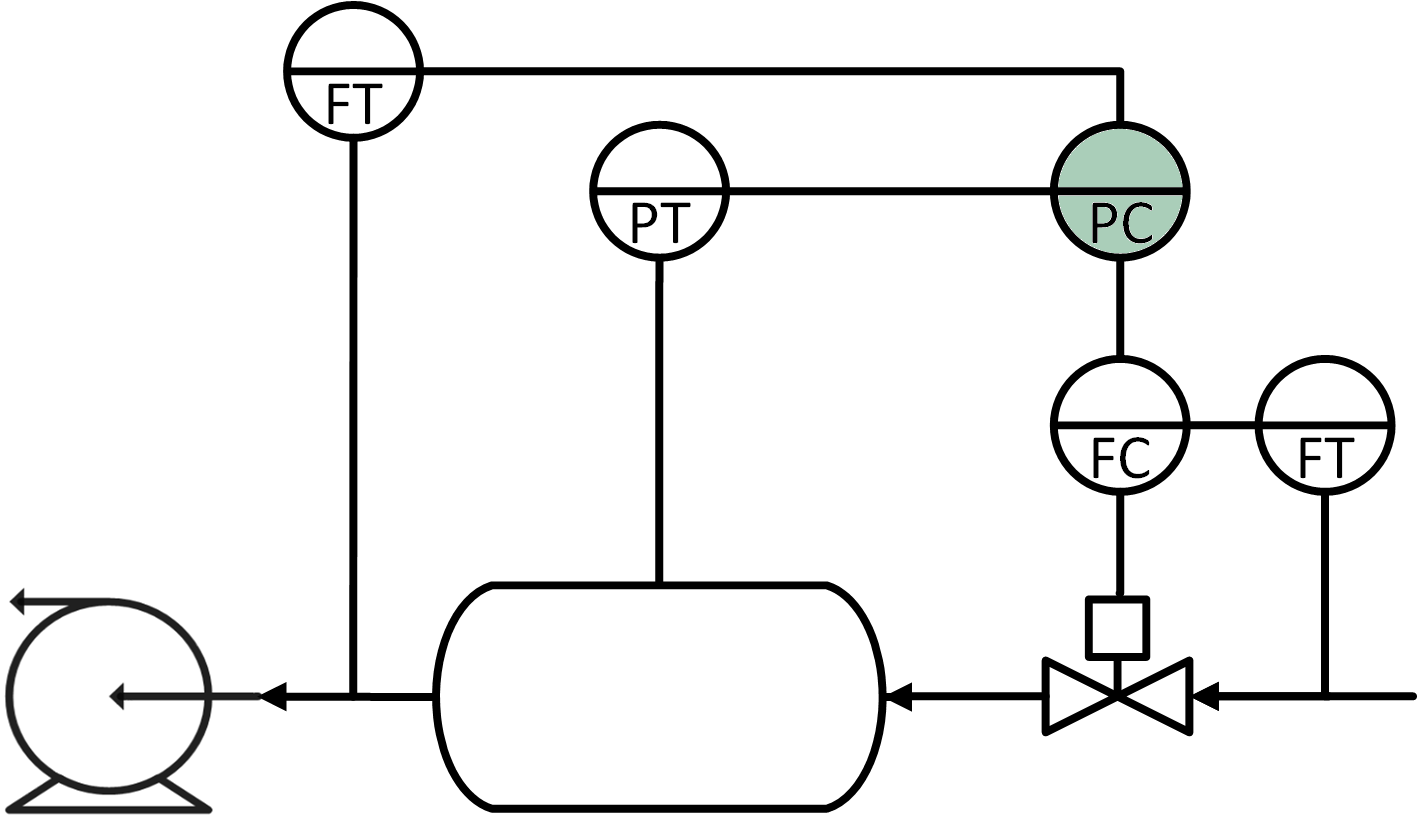## Quiz on Controller Design1. What element of control design best describes the demand for power from a dispatchable (coal, nuclear, natural gas, hydroelectric) power plant?

A. Controller set point
Correct. Dispatchable means that the power production can be adjusted to meet the demand. The demand is the set point for the power production. Wind and solar power are not dispatchable meaning that the level cannot be increased to meet an increased demand and shortfall in supply.
B. Disturbance
Incorrect. The demand does not directly affect how the power plant operates but is instead a signal on how much power should be generated. A disturbance for a power plant may be something like the ambient temperature that affects the cooling tower efficiency.
C. Actuator
Incorrect. An actuator is typically a valve, pump, motor, or other piece of equipment that makes physical change to the process.
D. Sensor
Incorrect. A sensor may detect a shortfall in demand from a frequency shift in the electrical grid. However, the demand is the target production level, not a sensor.

2. What is not a role of the Flow Controller (FC).A. Receives feedforward data about the inlet flow from a Flow Transmitter (FT)
Incorrect. The flow controller is a feedback controller with a feedforward flow input to anticipate the effect of inlet flow changes.
B. Adjusts the pressure of the tank based on reading from Pressure Transmitter (PT)
Correct. The role of the flow controller is to receive a flow rate set point from the pressure controller (PC) and adjust the valve to meet that target flow rate based on readings from the flow transmitter (FT). The Pressure Controller (PC) is the primary controller and not the other way around.
C. Adjusts the valve when not at a target flow rate
Incorrect. The flow controller actively adjusts the valve to meet a target flow rate.
D. Receives a set point from the Pressure Controller (PC).
Incorrect. The flow controller is a secondary controller that is commanded by the primary controller. In this case, the primary controller is the Pressure Controller (PC) that constantly updates a target flow rate set point.

3. If the feed forward Flow Transmitter (FT on the left) decreases, what is the correct action of the Pressure Controller (PC)?A. The Pressure Controller (PC) sends a signal to the valve to partially close.
Incorrect. The Pressure Controller (PC) gives a lower setpoint to the Flow Controller (FC). The Flow Controller (FC) will partially close the valve in response.
B. The Pressure Controller (PC) sends a lower set point to the Flow Controller (FC).
Correct. The tank will lose less pressure at a lower outlet flow rate and needs less inlet flow. The Pressure Controller (PC) sends a signal to the Flow Controller (FC) to partially close.
C. The Pressure Controller (PC) raises the tank pressure.
Incorrect. The pressure controller maintains a constant pressure set point. The Pressure Controller (PC) sends a signal to the Flow Controller (FC) to partially close to maintain this pressure.
D. The Pressure Controller (PC) lowers the tank temperature.
Incorrect. The Pressure Controller (PC) adjusts the pressure by changing the inlet flow set point.

4. What is the Controller Output (OP) and Process Variable (PV) for the Flow Controller (FC)?A. Inlet flow is the OP and Pressure is the PV.
Incorrect. The OP is the thing that is changed by the controller (valve). The PV is in the name of the controller (F=Flow) and also has the associated set point (SP).
B. The valve position is the PV and the inlet flow is the OP.
Incorrect. The valve position is the OP and the inlet flow is the PV.
C. Pressure is the OP and inlet flow is the PV.
Incorrect. The pressure is not part of this controller. It is only concerned with the inlet flow.
D. The valve position is the OP and the inlet flow is the PV.
Correct.

5. What is the Controller Output (OP) and Process Variable (PV) for the Pressure Controller (PC)?A. Inlet flow set point is the OP and Pressure is the PV.
Correct. The OP is the thing that is changed by the controller. In the case of this cascade control system, this is the set point to the secondary controller. The pressure is the PV and is measured to provide an increase for inlet flow when the pressure is too low.
B. The valve position is the PV and the inlet flow is the OP.
Incorrect.
C. Pressure is the OP and inlet flow is the PV.
Incorrect.
D. The valve position is the OP and the inlet flow is the PV.
Incorrect.